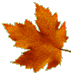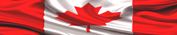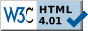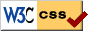# ELECTRONIC DESIGN NOTES - TRANSISTORSThe transistor was discovered in 1947 at Bell laboratories. Beginning in 1960s, the transistor takes on a spectacular ascending ramp in electronic design, and it continues to do so up to these very days! Even more, the future looks very bright for transistors, since we are moving now towards the "printed" electronic circuits--this means, electronic circuits printed on paper, and other materials, using special semiconductor/conductor inks.In this page are presented only a few instances of using transistors, some schematics, a few graphs, and a general classification. The structure employed is:

1. Biasing PNP and NPN BJT Transistors
2. BJT TRANSISTORS Functions
3. Biasing JFET Transistors
4. JFET Transistors Functions
5. Types of Transistors

NOTE
The basic notions highlighted in this page are related to a few electronic design topics presented in the first part, Hardware Design, of
LEARN HARDWARE FIRMWARE AND SOFTWARE DESIGN.1. BIASING PNP AND NPN BJT TRANSISTORSBipolar Junction Transistors (BJT)
work in two modes:

1. as amplifiers;
2. as digital switches in the saturation/cutoff states.

Many people do not understand this: bipolar transistors are current controlled electronic devices. Of course that we do need specific voltages to bias a bipolar transistor, except those voltages have the polarity, and the required magnitudes, according to the currents they need to generate.

That misunderstanding is nobody's fault, because there are very many books where this issue is unclear and/or incorrectly presented.

To start, let's analyze a few voltage biasing schematics, though please remember that all biasing voltages are generated by the needed currents. Transistors' graphs corresponding to the two functioning modes mentioned above are related to Ib, Ie, and Ic only, according to the formula:

Ie = Ib + Ic

Ie = emiter current
Ib = base current
Ic = collector current

To start, let's see how we saturate transistors, when they are used as logic components in the ON/OFF states. Please note: the saturation/cutoff digital states represent the only situation when transistors behave similar to voltage controlled relays. Unfortunately, this situation brings some confusion, therefore any analogy to "transistors' voltage control" should better be avoided.

 SATURATING BIPOLAR JUNCTION TRANSISTORS(IN DIGITAL MODE) Schematic Graph FigFig1: Saturating PNP transistors The red trace is the base voltage The blue trace is the voltage in point AThe graph is moved downwards for exemplification; the voltage variation is in fact from +5V to 0V. ATTENTION PNP transistors are saturated when the current Base-Emitter is at a maximum. However, due to the PNP special biasing requirements, that happens when base voltage is 0 V in the above picture. PNP transistors are in the cutoff state when the current Base-Emitter is at a minimum (zero). That happens when base voltage is the same as the emitter one--in the above example it is +5V. In the PNP biasing instance presented above, collector's voltage is a fixed value: zero volts.Fig 2: Saturating NPN transistors Red trace is the base voltageThe graph is moved downwards for exemplification; the voltage variation is in fact from 0V to +5V. Blue trace is the voltage in point A. Note that in this case collector's voltage is +12 V ATTENTION NPN transistors are saturated when the current Base-Emitter is at a maximum. However, due to the NPN biasing requirements, that happens when base voltage is greater than 0.7 V--otherwise, there is no current flow.NPN transistors are in the cutoff state when the current Base-Emitter is at a minimum (zero). That happens when the base voltage is the same as the emitter one--in the above example it is 0 V. In the NPN biasing case presented above, collector's voltage may have any positive value supported by the silicon. It can also be zero, although in that case we have no collector current.

As digital components in logic circuits, transistors are employed either as "control switches" or as "power drivers". Excellent working examples of both implementations are thoroughly presented in our LHFSD book.

Now, there are a few good methods to bias a BJT transistor when it works in "analog amplification mode", or in "digital/logic mode", as they are listed further down--though, only for the NPN transistor; for PNP you should reverse the polarities (somehow).

 BIASING ANALOG/LOGIC BIPOLAR JUNCTION NPN TRANSISTORS Schematic DescriptionFig 3: Base Biasing This schematic is very much dependant on transistor's β (Ic/IB). If β changes with the temperature, the output varies. The formulas used to calculate this schematic are: Ib = (+5V - Vbe) / Rb Ic = (+5V - Vbe) / (Rb / β) +5V is VcFig 4: Collector Feedback Biasing This schematic is very much similar to the one above.Fig 5: Universal Biasing This schematic is more stable when β changes, therefore it is also the most used one. The formulas employed to calculate this schematic are:  Ie = (Vre / Re)  Ie = (Vrb2 -Vbe) / Re  Ic = IeFig 6: Two Power Supply (Emitter Biasing) This schematic is dependant on the two voltage levels: if they are stable, so will be the output. The formulas used to calculate this schematic are:  Ib = (-5V - Vbe) / [Rb + (β+1) * Re]  Ie = (-5V - Vbe) / { [Rb / (β+1)] + Re}

NOTE

Always remember that a PNP transistor is biased according to the formula P-N-NN as follows:
1. Emitter = Positive (
P)
2. Base = Negative (
N)
3. Collector = More Negative than the Base (
NN)

NOTE
Always remember that an NPN transistor is biased according to the formula N-P-PP as follows:
1. Emitter = Negative (
N)
2. Base = Positive (
P)
3. Collector = More Positive than the Base (
PP)

The minimum amount of formulas needed to work with bipolar transistors are:

β (Beta) = Ic / Ib
α (Alpha) = Ic / Ie
Ie = (β + 1) * Ib
Ie = Ic + Ib

ATTENTION
The junction Base-Emitter is directly biased, while the junction Base-Collector is reverse biased. That means, there is current flowing from Base to Emitter (naturally), but there is (for certain) no current exchange between Base-Collector or Collector-Base.

For details about working with transistors in digital mode please consult LEARN HARDWARE FIRMWARE AND SOFTWARE DESIGN.

LEARN
HARD
FIRM
SOFT
DESIGN
NOW!2. BJT TRANSISTORS FUNCTIONS

The previously presented BJT saturation/cutoff states are specific to transistor's digital functionality. [That also defines BJT transistors as being perfect current controlled relays--which is one of their main functions.] Further, BJT transistors are considered DC logic elements, and they work as the very building bricks of all logic ICs (including processors).

It needs to be pointed out that BJT transistors have nicer switching characteristics compared to the MOS-FET ones. Even more, the Isolated Gate Bipolar Transistors (IGBT) have the nicest switching characteristics of all transistors--no ringing, or the minimum amount possible.

On the other hand, BJT transistors have been used since they were invented in analog circuits as amplifiers in linear mode. There are three main schematics employed to wire BJT transistors as analog amplifiers--they are presented, again, only for the NPN instance. What we are looking for is:

1. voltage gain
2. current gain
3. power gain

The three most common schematics employed are:

1. Common-Emitter, for voltage, current, and power gain
2. Common-Base, for voltage and power gain
3. Common-Collector, for current and power gain

 NPN TRANSISTORS - LINEAR ANALOG AMPLIFICATION TECHNIQUES Schematic Graph DescriptionFig 7: Simplified Common-Emitter amplifier The red trace is the In FM (Frequency Modulation) signal (transposed down) The blue trace is the amplified Out signal This schematic is used for:  1. voltage gain 2. current gain 3. power gain.Fig 8: Simplified Common-Base amplifier Red trace is the In FM (Frequency Modulation) signal (transposed down) Blue trace is the amplified Out signal This schematic is used for: 1. voltage gain 2. power gainFig 9: Simplified Common-Collector amplifier Red trace is the In FM (Frequency Modulation) signal (transposed down) Blue trace is the amplified Out signal This schematic is used for: 1. current gain 2. power gain

The article "Driving Automotive Injectors" [A25 in Amazing Articles] presents a few interesting topics about using power transistor drivers.3. BIASING JFET TRANSISTORS

FET (Field Effect Transistor) is a high-input impedance (100 MOhms and better), low noise, voltage controlled, solid-state semiconductor device. The first FET discovered was JFET (Junction Field Effect Transistor) followed a few years later by IGFET (Isolated Gate Field Effect Transistor) which was later renamed MOS-FET (Metal Oxide Semiconductor Field Effect Transistor).

The MOS technology is very cheap and perfectly suited for mass production, therefore it is used in most ICs today. For hardware designers, however, discrete FET components are rather expensive to procure, and they may be easily damaged by a simple hand touch (by our body-generated electrostatic voltage).

However, more problematic is biasing those FET transistors, therefore we present only a few common schematics.

ATTENTION
Before working with transistors (BJT or FET), you need to study their output curve. For that, you have to get their Data Sheets. Particularly to FETs, their output curve is fairly complex (not presented here). You need to get one, because it is possible there will be a few (unexplained) references to it.

ATTENTION
FET transistors behave similar to voltage controlled relays.

Three schematics are commonly employed to bias N-JFET transistors:

1. Self Biased
2. Universally Biased
3. Two Power Supply

 BIASING N-CHANNEL JFET TRANSISTORS Schematic DescriptionFig 10: Self Biased N-JFET We use the following formulas to calculate this schematic:  Id = Idss[1-(Vgs/Vgsoff)]2 Where Idss, and Vgsoff are given in the Data Sheet. Id = the Drain to Source current  Vrd = Id * Rd  Vrs = Id * Rs  Vd  = Vdd - Vrd  Vds = Vd - Vs  Vgs = Vg - VsFig 11: Universally Biased N-JFET We use the following formulas to calculate this schematic:  Id = Idss[1-(Vgs/Vgsoff)]2 Where Idss, and Vgsoff are given in the Data Sheet. In addition:  Vrg2 = (Vdd * Rg2) / (Rg2 + Rg1)  Vrd = Id * Rd  Vrs = Id * Rs  Vd = Vdd - Vrd  Vds = Vd - Vs  Vgs = Vg - VsFig 12: Two Power Supply Biased N-JFET We use the following formulas to calculate this schematic:  Id = Idss[1-(Vgs/Vgsoff)]2 Where Idss, and Vgsoff are given in the Data Sheet. In addition:  Vrd = Id * Rd  Vrs = Id * Rs  Vd = Vdd - Vrd  Vds = Vd - Vs  Vgs = Vg - Vs

A practical method of working with FET transistors is, always test their output curve before using them. FETs are "third order semiconductors", and it is not easy to control and to calculate them. First, decide on using one or two FET functions, for example "switching" and/or "variable resistor", and then use a simulator program, or a test stand to discover the right values need for the proper biasing resistors.

The test stand looks (generally, in principle) as follows:Fig 13: Test stand used to discover the right biasing resistors required by various FET configurations Once you calculate and measure the needed voltages and currents, you should implement one of the above biasing schematics. It is easy to find the right gate voltage/resistor using:  R = V / I
LHFSD5
SC ED5RV07

4. JFET TRANSISTORS FUNCTIONS

All FET transistors have three main functions. They are used as:

1. amplifiers
2. analog/digital switches
3. voltage-controlled resistors

If you want digital FET switches/relays in your application, please consider one of the biasing schematics presented above. The voltage-controlled resistor function is left for you to discover. Further is presented only the amplification function.

 N-CHANNEL JFET TRANSISTORS - AMPLIFICATION TECHNIQUES Schematic Graph FigFig 14: Common Source JFET amplifier Red trace is the In FM (Frequency Modulation) signal (transposed down) Blue trace is the amplified Out signalFig 15: Common Drain JFET amplifier Red trace is the In FM (Frequency Modulation) signal (transposed down) Blue trace is the amplified Out signalFig 16: Common Gate JFET amplifier Red trace is the amplified Out signal Blue trace is the In FM (Frequency Modulation) signal (transposed down)5. TYPES OF TRANSISTORS

It is possible transistors are the electronic components coming in the wildest variety possible (this is, considering their technical characteristics, not their shape which is standard), and that clearly indicates they are very much used. Above are presented only the BJT and the JFET ones. More or less, all other existing types of transistors are similar in functionality.

It is possible we will attempt to develop this "Types of transistors" topic one glorious day. Meanwhile, we do encourage you to read
LEARN HARDWARE FIRMWARE AND SOFTWARE DESIGN. In addition to presenting all the schematics you need to start working with dsPIC controllers (or with any other type of Microchip controller), this book presents 12 firmware plus 7 software Source-Code applications, each of them being a practical working project, fairly easy to understand. [These Source Code applications are priceless!]

Please believe this: complete, working, and thoroughly explained firmware Source Code programs in a book is unheard of! Even more, the really exceptional aspect is, all firmware and software programs presented in LHFSD are the essence of simplicity, and they are truly logic. Always remember that it is the firmware/software that drives the hardware.Take control of this extraordinary IT Revolution with Learn Hardware Firmware and Software Design--the easy way!» LINK TO "LOGICALLY STRUCTURED ENGLISH GRAMMAR"Page last updated on: July 25, 2018Site pages valid according to W3CStylesheets pages valid according to W3C